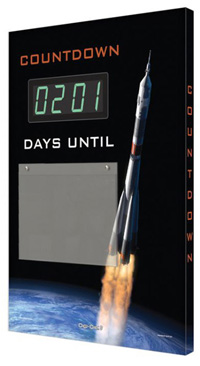MSDSTopics Free Sites FAQ's Regulations Glossary Software Suppliers Books Forum Poll Fun stuff Quiz Store Understand your MSDS with the MS-Demystifier Search ALL our MSDS info

Energy Unit Conversions

### Definition

Here is a handy conversion calculator for some common energy, wavelength and frequency terms, including some that you might find on an Safety Data Sheet.

 Energy Conversion Calculator(Enter number on the left side; answer appears on the right side) This many: cm-1kJ/molkcal/molnmHzKHzMHzGHzTHzeVHartree Equals cm-1kJ/molkcal/molnmHzKHzMHzGHzTHzeVHartreeHelp your employees understand control of hazardous energy with safety banners and lockout/tagout supplies from Safety Emporium.

Energy can be expressed many ways. Two relationships allow us to freely convert between energy, wavelength and frequency (see below). If we know any one of these three properties, we know the other two.

Wavenumber
(cm-1)
A wavelength of energy that is also called a reciprocal centimeter. Wavenumbers are obtained when frequency is expressed in Hertz and the speed of light is expressed in cm/s. This unit is commonly used in infrared spectroscopy.
Kilojoules per mole
(kJ/mol, kJ.mol-1)
A Joule, J, is the SI unit of energy and is defined as one kg.m2/s2. The prefix "kilo" means 1,000, so one kJ = 1,000 J. As the energies associated with a single molecule or atom are quite small, we often find it easier to discuss the energy found in one mole of the substance, hence "per mole". To get the energy for one molecule, divide kJ/mol by Avogadro's number, 6.022 x 1023.
Kilocalories per mole
(kcal/mol, kcal.mol-1)
A calorie was originally defined as the amount of energy required to raise the temperature of one gram of water by one degree Celsius. One calorie = 4.184 J. One kcal = 1,000 cal. When we count calories in our food, we are actually referring to kilocalories; e.g. 1 dietary Calorie = 1,000 cal = 1 kcal. See the note in the previous entry for information about the mole part of this unit.
Nanometer
nm
The prefix "nano" means 1 x 10-9 = 0.000000001 = 1/1,000,000,000. Therefore, a nanometer refers to energy with a wavelength that is 1/1,000,000,000th of a meter. Visible light is made of up electromagnetic radiation that has wavelengths ranging from roughly 300 to 800 nm. For additional conversions of nm (as a distance, not energy), see our distance units page.
Hertz
(s-1, Hz, /s)
A Hertz is a unit of frequency defined as a reciprocal second, s-1. For example, AC current cycles polarity 60 times per second, so we could call this 60 Hz = 60 s-1. Human hearing has a frequency range from a few hundred Hz up to approximately 20,000 Hz (20 KHz).
Kilohertz
(kHz)
The prefix "kilo" means 1,000, so there are 1,000 Hz in one kHz. Likewise, there are 1,000 kHz in one MHz.
Megahertz
(MHz)
The prefix "mega" means 1,000,000, so there are 1,000,000 Hz = 1,000 kHz in one MHz. This is a typical frequency for radio equipment as well as high-tech scientific instruments such as magnetic resonance imaging (MRI, or NMR) scanners.
Gigahertz
(GHz)
The prefix "giga" means 1,000,000,000, so there are 1,000,000,000 Hz = 1,000,000 KHz = 1,000 MHz in one GHz. This is a typical frequency domain for high speed short-range wireless networks
Terahertz
(GTHz)
The prefix "tera" means 1,000,000,000,000, so there are 1,000,000,000,000 Hz = 1,000,000,000 KHz = 1,000,000 MHz = 1,000 GHz in one THz. THz scanners have recently found use in airport passenger screening devices.
Electron Volt
(eV)
The electron volt is the energy that we would give an electron if it were accelerated by a one volt potential difference. 1 eV = 1.602 x 10-19 J. This term is most often used by physicists and electrochemists.
Hartree
(Eh or Ha)
A hartree (or the Hartree Energy) is the atomic unit of energy and is defined as 2Rhc, where R is the Rydberg constant, h is Planck's constant c is the speed of light in a vacuum. This is clearly not something you'd find on an SDS.

For the budding scientist: Here are the two relationships that relate energy, frequency and wavelength. The first is c = νλ where c = the speed of light in vacuum (3.00 x 108 m/s), ν = frequency and λ is wavelength. The second is E = hν where E = energy, h is a special constant called Planck's constant (6.63 x 10-34J.s) and ν is again frequency. The second equation tells us that frequency is proportional to energy. Combining the two, we find that frequency and energy are inversely proportional to wavelength.This electronic event countdown sign and other safety scoreboards from Safety Emporium can keep your safety program on course.

### SDS Relevance

Appendix D of OSHA Hazard Communication Standard does not specify English or metric units. Therefore, be very careful to note the units when reading numbers such as temperature or pressure units on a Safety Data Sheet.

If you ever perform a conversion or calculation of any sort, always remember to write the units next to each number in your calculation and make sure that they cancel properly. NASA once lost a \$125 million space probe because of a (failure to convert) unit error! See the last two links below under Further Reading for more on unit conversions.